﻿

### Characterization of idempotent 2-copulas

#### Abstract

A 2-copula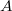$A$ induces a transition probability function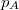$p_A$ via

where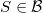$S\in \cal B$,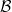$\cal B$ denoting the Lebesgue measurable subsets of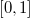$[0,1]$.  We say that a set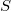$S$ is invariant under$A$ if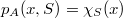$p_A(x,S)=\chi _S(x)$for almost all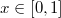$x\in [0,1]$,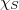$\chi _S$ being the characteristic function of$S$.  The sets$S$ invariant under$A$ form a sub-$\sigma$-algebra of theLebesgue measurable sets, which we denote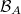${\cal B}_A$. A set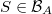$S\in {\cal B}_A$is called an atom if it has positive measure and if for any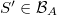$S'\in {\cal B}_A$,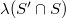$\lambda (S'\cap S)$ is either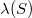$\lambda (S)$ or 0.
A 2-copula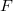$F$ is idempotent if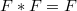$F*F=F$. Here$*$ denotes the product defined in . Idempotent 2-copulas are classified and characterized asfollows:
(i) An idempotent$F$ is said to be nonatomic if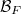${\cal B}_F$ contains noatoms.  If$F$ is a nonatomic idempotent, then it is the product of a leftinvertible copula and its transpose.  That is, there exists a copula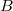$B$ such that
where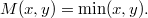$M(x,y)=\min(x,y).$
(ii) An idempotent$F$ is said to be totally atomic if there exist essentiallydisjoint atoms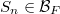$S_n\in {\cal B}_F$ with
If$F$ is a totally atomic idempotent, then it is conjugate to an ordinal sumof copies of the product copula.  That is, there exists a copula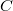$C$ satisfying$C*C^T=C^T*C=M$ and a partition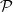$\cal P$ of$[0,1]$ such that
\begin{equation}F=C*(\oplus _{\cal P}F_k)*C^T \end{equation} where eachcomponent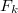$F_k$ in the ordinal sum is the product copula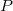$P$.
(iii) An idempotent$F$ is said to be atomic (but not totally atomic) if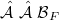${\cal B}_F$ contains atoms but the sum of the measures of a maximal collection ofessentially disjoint atoms is strictly less than 1.  In this mixed case, thereexists a copula$C$ invertible with respect to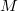$M$ and a partition$\cal P$ of$[0,1]$ for which (1) holds, with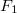$F_1$ being a nonatomic idempotent copula andwith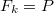$F_k=P$ for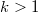$k>1$.
Some of the immediate consequences of this characterization are discussed.

DOI Code: 10.1285/i15900932v30n1p147

Keywords:
copula; idempotent; star product

Classification: 60G07; 60J05; 60J25

Full Text: PDF